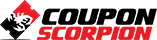# Financial Statement Analysis Ratio Analysis

Horizontal analysis, vertical analysis, ratio analysis-liquidity & efficiency, solvency, profitability, & market ratios

### What you’ll learn

• List and describe the tools for financial statement analysis
• Understand, calculate, and analyze horizontal analysis
• Explain, calculate and analyze vertical analysis
• List and describe the categories of ratio analysis
• Describe liquidity ratios
• Examine solvency ratios
• Explain profitability ratios
• Discuss market prospects ratios
• Calculate and analyze current ratio, acid-test ration, and accounts receivable ratio
• Analyze and compute inventory turnover ratio, days sales uncollected, days sales in inventory, and total asset turnover
• Discuss and calculate debt ratio, equity ratio, debt-to-equity ratio, and times interest earned
• Explain and compute profit margin ratio, gross margin ratio, and return on total assets
• Describe and calculate return on equity, book value per common share, and earnings per share
• Calculate and analyze price earnings ratio and dividend yield
• Discuss and analyze the components of a financial statement analysis report

### Requirements

• Understanding of basic accounting terms

### Description

Learn tools to analyze financial statements, including vertical analysis, horizontal analysis, and ratio analysis.

This course will provide resources such as downloadable PDF files and preformatted Excel spreadsheet to help understand and apply the concepts.

We will list and describe the tools for financial statement analysis, including vertical analysis, horizontal analysis, and ratio analysis.

Below is more detail about the concepts we will learn.

This course will help us understand, calculate, and analyze horizontal analysis. Horizontal analysis is used to compare financial data and performance of a company across time and is a common and intuitive form of analysis.

We will explain, calculate, and analyze vertical analysis. Vertical analysis also termed common size financial statements, displays all items as percentages of a common base figure instead of absolute numbers. Vertical analysis is very useful to compare financial statements for companies of different sizes.

The course will list and describe the categories of ratio analysis. Ratio analysis is a common form of financial statement analysis used to obtain a quick indication of a businesss financial performance in different areas. Ratios are often grouped into categories, including liquidity ratios, solvency ratios, profitability ratios, and market prospects ratios.

We will describe liquidity and efficiency ratios. We will list liquidity and efficiency ratios, perform calculations, and analyze their use.

The course will examine solvency ratios, listing solvency ratios, calculating solvency ratios, and analyzing their use.

We will Explain profitability ratios. The course will list profitability ratios. We will perform calculations and analysis of profitability ratios.

We will discuss market prospects ratios, listing out the market prospect ratios, discussing the formulas, and analyzing the use of market prospect ratios.

The course will calculate and analyze current ratios, acid-test ration, and accounts receivable ratios.

We will analyze and compute inventory turnover ratios, days sales uncollected, days sales in inventory, and total asset turnover.

The course will discuss and calculate the debt ratios, equity ratios, debt-to-equity ratios, and times interest earned.

We will explain and compute the profit margin ratio, gross margin ratio, and return on total assets.

The course will describe and calculate return on equity, book value per common share, and earnings per share.

We will calculate and analyze price earnings ratio and dividend yield.

The course will discuss and analyze the components of a financial statement analysis report. We will explain what software can do for us and what software cannot do.

Author(s): Robert (Bob) Steele

Deal Score+4
\$12.99 \$79.99